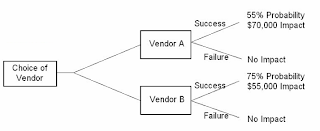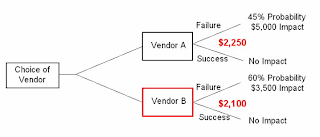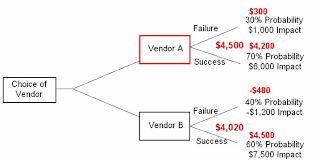## Saturday, February 16, 2013

### Decision Tree Analysis

In the previous chapter, we took a look at Expected Monetary Value or EMV Analysis. The Decision Tree Analysis is another tool/technique that we use in Quantitative Risk Analysis that directly uses this EMV Analysis. In this chapter, we are going to take a detailed look at Decision Tree Analysis

Decision Tree Analysis

Decision Tree Analysis is used to make decisions based on the risks that could impact us in the various possible scenarios we may encounter in future. It calculates the Expected Future Value of an activity based on the current impact & probability of all risks.

Decision Tree Analysis uses a Decision Tree Diagram. In the tree, we start at the starting point and go through the tree and take a decision based on the EMV for the Alternatives that are available for us. It shows a sequence of inter-related decisions and their respective EMVs so that you can take a good and properly thought-out decision. Decision Tree Analysis is used typically to take decisions dealing with Time or Cost.

Let us now take a look at some examples to understand Decision Tree Analysis:

Example 1:

Let us say, we are given the task of deciding between Vendor A and Vendor B. Vendor A has a Success Probability of 55% and an Impact of \$ 70,000 while there is no impact on Failure. Similarly Vendor B has a 75% probability of Success and has an impact of \$ 55,000 and he too has no impact on Failure. Based on this information, how would you choose the Vendor?

The simple Answer would be – Use Decision Tree Analysis. So, based on this question, if I were to create a Decision Tree, it would look like below:So, here:

EMV for Vendor A: = 70000 * 55% = 38,500

EMV for Vendor B = 55000 * 75% = 41,250Now, you know the EMV for each vendor. So, the wiser choice would be to choose Vendor B because the Expected Monetary Value of choosing Vendor B is greater than Vendor A.

Trivia:
If we had just considered the Impact, Vendor A would look like a better choice because he has a higher impact. But, he has a lower probability. So, Vendor B, even though has a lower impact, is selected because the combination of both probability and impact makes him the better choice.

Example 2:

Example 1 was an all positive scenario where there is no Impact for failure. What would you do in an all Negative Scenario?? Look at the Decision Tree Below:In this example, in both cases, there is no impact if the outcome is a success. But, both Vendors A & B have an impact on failure and have a probability of failure too. So in this case, you may be wondering why I chose Vendor B instead of A, even though the EMV for A is higher. Are you???

In example 1, we were looking at positive EMV (For Success or Profits) so, we chose the vendor with higher profitable EMV. Whereas, in this case we are calculating Negative EMV (For Failure or Losses). So, choosing the vendor who would cause lower losses in case of a failure would be a better choice. Wouldn’t it??

Example 3: In examples 1 & 2, we took a look at trees that either had a positive or negative impact only. What must we do if we have both? Look at the tree below:In this case, both Vendors A & B have Impact on both Success & Failure. So, the EMV for each vendor is the sum of the Individual EMV’s.

For Vendor A:

EMV for Failure = 1000 * 30% = 300

EMV for Success = 6000 * 70% = 4200

Total EMV for Vendor A = \$ 4,500/-

For Vendor B:

EMV for Failure = -1200 * 40% = -480

EMV for Success = 7500 * 60% = 4500

Total EMV for Vendor B = \$ 4,020/-

So, based on the total EMV, Vendor A is the better choice…

Trivia:
Did you note that Vendor B has a negative monetary impact in case of failure??? Be careful and note the – sign… If you did the calculation in a hurry and ignore the – symbol, your EMV for Vendor B would’ve been \$ 4,980 suggesting that Vendor B is the better choice. Whereas, the truth was that, because of the –ve impact on failure, Vendor A is the better choice.
Example 4:

In all of the examples above, our decision was based solely on the impact and probability of the scenario’s outcome. What must we do in cases where the decision should also take into account the initial expenses incurred for the activity?

Let’s say, your company has grown hugely in the past couple of years and your current office does not have enough space to accommodate the new guys. So, now you have two choices – Either to construct/purchase a new office or expand the current premises to accommodate the newer guys. In either case, there is cost involved in completion of the office premises. Plus, there could be a high demand for the new space which results in high profits or there could be a low demand resulting in lower profits. This scenario is outlined in the Decision Tree below:In Tree’s where an initial investment is present, we proceed just like the other scenarios wherein we calculate the EMV for each alternative and sum it up. After that, we deduct the Initial Expenses to arrive at the actual monetary value of the alternative.

Trivia:
In simpler terms, let’s say, I invest 5,000 rupees today and earn 10,000 after 6 months, my profit is 5,000 whereas, if I invest 10,000 rupees and earn 12,000 at the end of 6 months, my profit is only 2,000. Though the eventual money I get at the end of 6 months is higher in the second case, it also means that I invest a larger amount up front thereby reducing profits. So, option 1 where in invest 5000 and get 10000 at the end of 6 months is the better choice. Isn’t it?

So, the EMV Calculation works out as follows:For Build:

Total EMV = 522,000

If we include Initial Cost – Net EMV = \$ 292,000/-

For Expand:

Total EMV = 393,000

If we include Initial Cost – Net EMV = \$ 298,000/-

So, the decision here would be to expand the current office premises. Even though the profits that we may earn if we move to a new office are higher, there is a higher impact if people are not willing to move and a high initial cost. As a result, the EMV of expanding the current office is more profitable and hence it is selected.

Some Important Decision Tree Related Terms:

For the exam, actually speaking, whatever we have covered so far is more than sufficient. But, for the same of completeness, I want to cover one last topic related to Decision Trees. There are 3 key terms that we will use while using Decision Tree’s in real life. They are:

a. Decision Node – The Point where an action or decision needs to be made – Signified by a solid black square
b. Chance Node – The Point where events that cannot be controlled by the person who is taking the decision happen – Signified by a solid black dot
c. End of Branch - The end point with nothing connected on one end – Signified by a solid tilted Triangle.

The sample Decision Tree below can help you understand better – as to what I am trying to convey here:I repeat, knowing these terms or these symbols are not required for the Exam. But, we are not studying just to become certified. Our aim is to become better Risk Managers. So, knowing these will only help you perform your duties better…

Prev: Expected Monetary Value Analysis

Next: Modeling and Simulation

1.2.great thank you sir

3.i have a one problem to solve by decision tree analysis. may i get you help.

1.4.Extremely well explained

© 2013 by www.getpmpcertified.blogspot.com. All rights reserved. No part of this blog or its contents may be reproduced or transmitted in any form or by any means, electronic, mechanical, photocopying, recording, or otherwise, without prior written permission of the Author.